# 挖掘算法中的数据结构（五）：排序算法总结 和 索引堆及优化（堆结构）

• 排序算法总结
• 索引堆及其优化
• 堆结构衍生的问题

## 一. 排序算法总结（1）均时间复杂度

（2）原地排序

（3）额外空间

• 对于插入排序和堆排序而言，使用的额外空间就是数组上交换元素，所以所耗空间为O(1)级别，即常数级别。
• 而归并排序需要O(n)级别空间，即数组同等长度空间来辅助完成归并过程。
• 快速排序所需O(logn)额外空间，因为它采用递归方式来进行排序，递归有logn层，所以需要O(logn)空间来保证每一层的临时变量以供递归返回时继续使用。

（4）稳定排序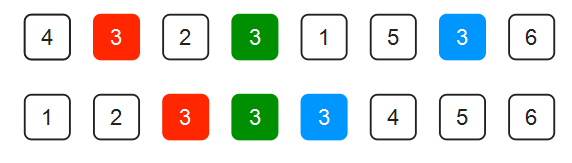• 稳定排序
• 插入排序：算法中有后面元素与前面元素相比较，若小于则前移，否则不动。所以相同元素之间位置不会发生改变。
• 归并排序：在归并过程中，左右子数组已经有序，需要归并到一起，其核心也是判断当后面元素小于前面元素才前移，否则不动。所以相同元素之间位置不会发生改变。
• 不稳定排序
• 快速排序：算法核心中会随机选择一个标志点来进行大于、小于判断排序，所以很有可能使得后面相等元素到前面来。所以相同元素之间位置会发生改变。
• 堆排序：将整个数组整理成堆的过程中会破坏掉稳定性。所以相同元素之间位置会发生改变。

## 二. 索引堆（Index Heap）及优化

### 1. 引出问题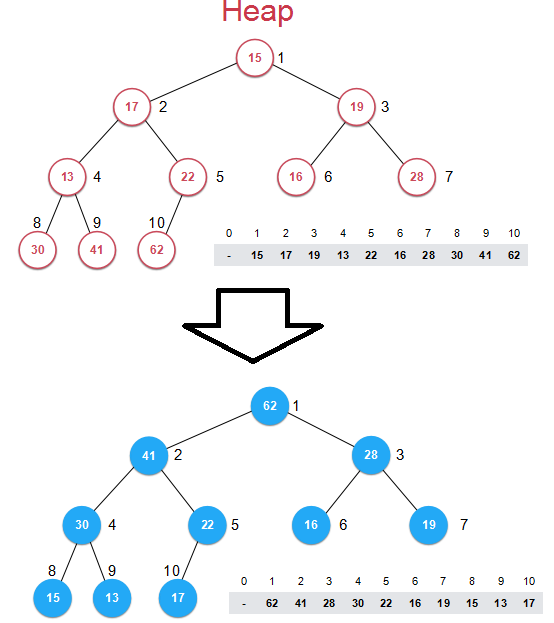• 如果元素是非常复杂的结构，例如字符串（一篇十万字的文章）等等，这样交换的代价是十分大的。不过这可以通过一些基本手段解决，
• 更加致命的是元素在数组中的位置发生改变，使得在堆中很难索引到它！例如元素下标是任务ID，元素值是优先级别。当将数组构建成堆后，下标发生改变，则意味着两者无法产生联系！在原来数组中寻找任务只需O(1)，但是构建成堆后元素位置发生改变后需要遍历数组！所以才会引入“索引堆”这个概念。

### 2. 结构思想

• 当将此数组构建成堆之前：对于索引堆来说将数据和索引两部分内容分开存储，而真正表示堆的数组是由索引构建成的，如下图，每一个节点旁标记的是索引1,2,3……

• 当将此数组构建成堆之后：****data部分并未发生改变，真正改变的是索引index，index数组发生改变形成堆。index为10，即真正元素值去找索引10代表的data值62，这样去对应。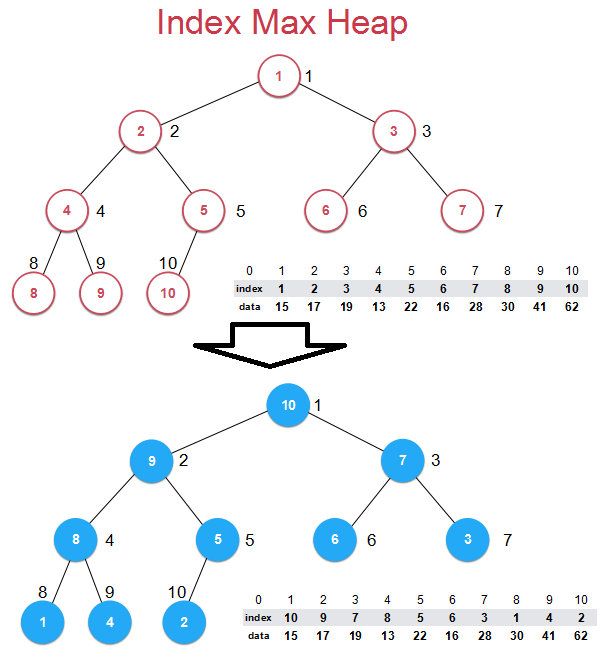• 将数组构建成堆之后，只是索引index值发生改变，int型数字之间的交换而不会涉及到data数据类型，提供交换效率。
• 重要的一点，如果想对堆中数据进行操作，例如对index为7的数据进行修改，找到对应数据值为28更改，在修改之后需要继续维护“堆”的性质，这时只需对data数组进行维护即可。

### 3. 代码实现

（1）基本更改

• 在成员变量中需要添加一个数组来存储索引值
• 在构造函数中需要开辟索引数组空间
• 在析构桉树中也要相信释放掉该空间

（2）插入和删除函数

• 插入函数：在调用插入函数时不仅要传数据还要传递索引值。注意：这里获取data数组的索引值是根据index数组来确定的！在shiftUp函数中交换的是index索引值，并非是data数组。
    // 索引堆中, 数据之间的比较根据data的大小进行比较, 但实际操作的是索引
void shiftUp( int k ){
while( k > 1 && data[indexes[k/2]] < data[indexes[k]] ){
swap( indexes[k/2] , indexes[k] );
k /= 2;
}
}

// 向最大索引堆中插入一个新的元素, 新元素的索引为i, 元素为item
// 传入的i对用户而言,是从0索引的
void insert(int i, Item item){
assert( count + 1 <= capacity );
assert( i + 1 >= 1 && i + 1 <= capacity );

i += 1;
data[i] = item;
indexes[count+1] = i;
count++;

shiftUp(count);
}
• 删除函数：
    // 索引堆中, 数据之间的比较根据data的大小进行比较, 但实际操作的是索引
void shiftDown( int k ){

while( 2*k <= count ){
int j = 2*k;
if( j + 1 <= count && data[indexes[j+1]] > data[indexes[j]] )
j += 1;

if( data[indexes[k]] >= data[indexes[j]] )
break;

swap( indexes[k] , indexes[j] );
k = j;
}
}

// 从最大索引堆中取出堆顶元素, 即索引堆中所存储的最大数据
Item extractMax(){
assert( count > 0 );

//注意：这里获取data数组的索引值是根据index数组来确定的
Item ret = data[indexes];
swap( indexes , indexes[count] );
count--;
shiftDown(1);
return ret;
}

（3）更改函数

   // 将最大索引堆中索引为i的元素修改为newItem
void change( int i , Item newItem ){

i += 1;
data[i] = newItem;

// 找到indexes[j] = i, j表示data[i]在堆中的位置
// 之后shiftUp(j), 再shiftDown(j)
for( int j = 1 ; j <= count ; j ++ )
if( indexes[j] == i ){
shiftUp(j);
shiftDown(j);
return;
}
}

### 4. 反向查找优化 —— 更改元素值

（1）引出问题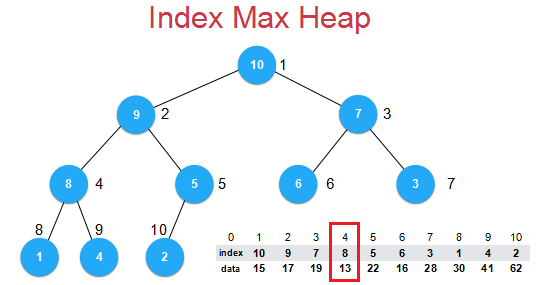（2）思想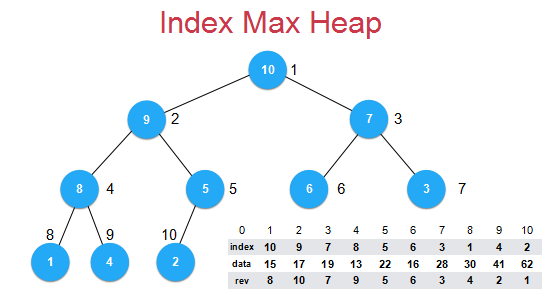rev数组相关性质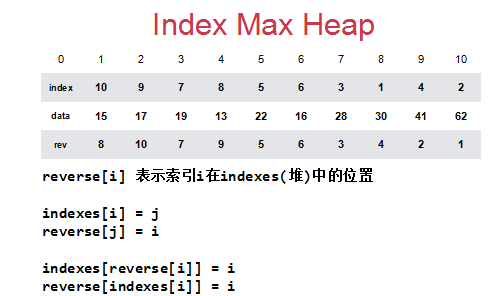（3）代码实现

（具体代码见github源码，以下只粘贴重点部分）

    int *reverse;   // 最大索引堆中的反向索引, reverse[i] = x 表示索引i在x的位置

// 索引堆中, 数据之间的比较根据data的大小进行比较, 但实际操作的是索引
void shiftUp( int k ){

while( k > 1 && data[indexes[k/2]] < data[indexes[k]] ){
swap( indexes[k/2] , indexes[k] );
reverse[indexes[k/2]] = k/2;
reverse[indexes[k]] = k;
k /= 2;
}
}

// 索引堆中, 数据之间的比较根据data的大小进行比较, 但实际操作的是索引
void shiftDown( int k ){

while( 2*k <= count ){
int j = 2*k;
if( j + 1 <= count && data[indexes[j+1]] > data[indexes[j]] )
j += 1;

if( data[indexes[k]] >= data[indexes[j]] )
break;

swap( indexes[k] , indexes[j] );
reverse[indexes[k]] = k;
reverse[indexes[j]] = j;
k = j;
}
}

// 向最大索引堆中插入一个新的元素, 新元素的索引为i, 元素为item
// 传入的i对用户而言,是从0索引的
void insert(int i, Item item){
assert( count + 1 <= capacity );
assert( i + 1 >= 1 && i + 1 <= capacity );

// 再插入一个新元素前,还需要保证索引i所在的位置是没有元素的。
assert( !contain(i) );

i += 1;
data[i] = item;
indexes[count+1] = i;
reverse[i] = count+1;
count++;

shiftUp(count);
}

// 从最大索引堆中取出堆顶元素, 即索引堆中所存储的最大数据
Item extractMax(){
assert( count > 0 );

Item ret = data[indexes];
swap( indexes , indexes[count] );
reverse[indexes[count]] = 0;
reverse[indexes] = 1;
count--;
shiftDown(1);
return ret;
}

// 将最大索引堆中索引为i的元素修改为newItem
void change( int i , Item newItem ){

assert( contain(i) );
i += 1;
data[i] = newItem;
// 有了 reverse 之后,
// 我们可以非常简单的通过reverse直接定位索引i在indexes中的位置
shiftUp( reverse[i] );
shiftDown( reverse[i] );
}

## 五. 堆衍生的问题

### 1. 使用堆实现优先队列

（1）OS系统执行任务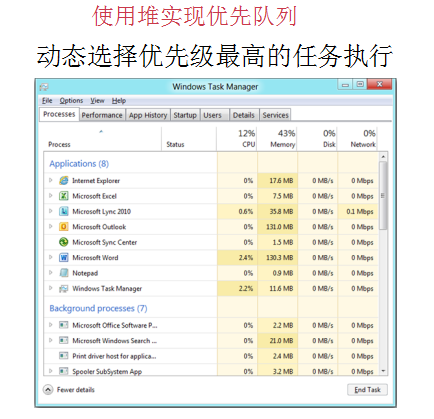（2）在N个元素中选出前M个元素

### 2. 多路归并排序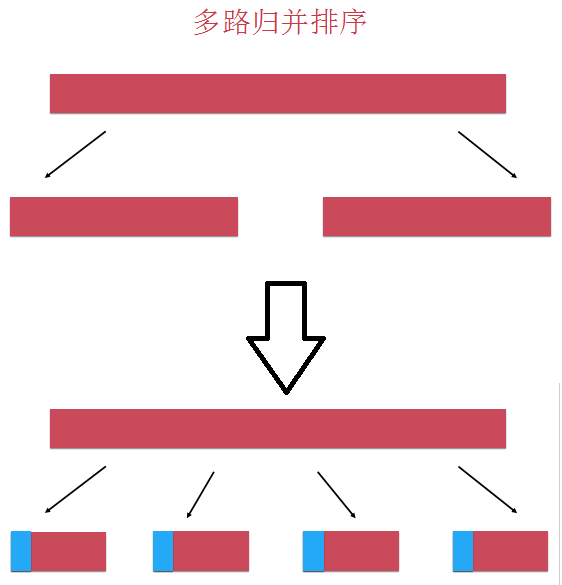### 3. d叉堆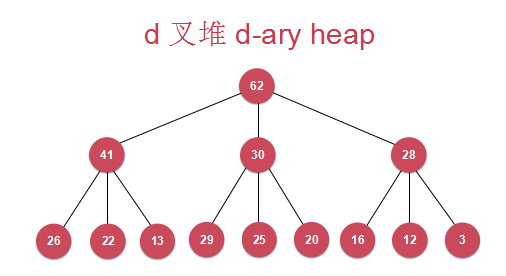### 4. 堆的实现细节优化

• ShiftUp 和 ShiftDown 中使用赋值操作替换swap操作
• 表示堆的数组从0开始索引
• 没有capacity的限制，动态的调整堆中数组的大小

### 5. 其它

https://github.com/liuyubobobo/Play-with-Algorithms

04-09496
05-1637709-15695
07-25318
02-14625
08-222515
08-171197
03-13186
08-141468
05-0879